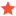Problems drawing arcabenedikAdministratorPosts: 542 Threads: 8 %%TYL_NUMTHANKEDLIKED%% Joined: Sep 2009 Reputation: 4 02-12-2020, 10:32 AM The ZeroAngleDirection property defines the Vector3D that specifies the 3D direction where the angle is zero. So if StartAngle is 0, then the arc will start in the direction of ZeroAngleDirection  (CenterPosition + ZeroAngleDirection * Radius * HorizontalStretchFactor). The direction of the arc is in the clockwise direction - from StartAngle to EndAngle. For example the arc from the Ab3d.PowerToys samples is defined as Code:`````` Here the normal points up and the ZeroAngleDirection points in the x axis direction (let's say that this is to the right). The StartAngle is set to 0 so this means that the arc will start at the right side. Its EndAngle is set to 270. So this means that the arc will be drawn in the clockwise direction from 0 to 270 degrees - so from the right, then to the bottom (z direction), to the left and end on top (in -z direction) showin 3/4 of the circle. Here is the source of the code that defines the arc positions: Code:```       public static void AddArc3DPoints(Point3D circleCenterPosition,                                          Vector3D circleNormal,                                          Vector3D zeroAngleDirection,                                          double xRadius, // radius at zero angle direction                                          double yRadius,                                          double startAngle,                                          double endAngle,                                          int segments,                                          Point3DCollection points)        {            if (startAngle == endAngle)                return;            circleNormal.Normalize();            zeroAngleDirection.Normalize();            var rightDirection = Vector3D.CrossProduct(zeroAngleDirection, circleNormal);            var angleStep = ((endAngle - startAngle) * Math.PI / 180) / segments;            var angle = startAngle * Math.PI / 180;            for (int i = 0; i <= segments; i++)            {                // Get the position in 3D based on the orientation of the circle (circleNormal, zeroAngleDirection)                var onePosition = circleCenterPosition;                onePosition += Math.Sin(angle) * yRadius * rightDirection;                onePosition += Math.Cos(angle) * xRadius * zeroAngleDirection;                // Add position                points.Add(onePosition);                if (i == segments - 1)                    angle = endAngle * Math.PI / 180; // this prevents double inprecission                else                    angle += angleStep;            }        }``` Andrej Benedik Find Reply

 Messages In This Thread Problems drawing arc - by Radu Iordanescu - 02-11-2020, 03:43 PM RE: Problems drawing arc - by abenedik - 02-12-2020, 10:32 AM RE: Problems drawing arc - by Radu Iordanescu - 02-12-2020, 06:33 PM RE: Problems drawing arc - by abenedik - 02-12-2020, 11:35 PM

Forum Jump:

 Users browsing this thread: 1 Guest(s)